Thermodynamics and Propulsion

# 14.2 The Rocket Equation

We can now look at the role of specific impulse in setting the performance of a rocket. A large fraction (typically 90%) of the mass of a rocket is propellant, thus it is important to consider the change in mass of the vehicle as it accelerates.There are several ways to do this through applying conservation of momentum. Here we will apply the momentum theorem differentially by considering a small mass,, expelled from the rocket during time, Figure 14.2.

The initial momentum of the mass in the control volume (the vehicle) is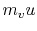. The final momentum of mass in the control volume (the vehicle and the mass expelled,) is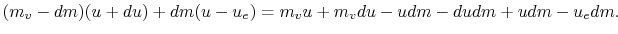The change in momentum during the intervalis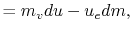sinceis a higher order term. Now consider the forces acting on the system which is composed of the masses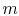(the rocket), and(the small amount of propellant expelled from the rocket during time):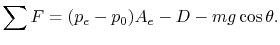Applying conservation of momentum, the resulting impulse,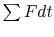, must balance the change in momentum of the system: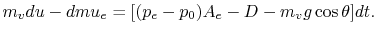Then since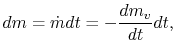whereis the propellant mass flow rate, we have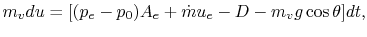or, for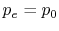,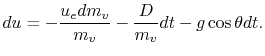(14..1)

Equation 14.1 is known as The Rocket Equation. It can be integrated as a function of time to determine the velocity of the rocket.

If we set, assume that at,, neglect drag, and set, then we can simplify the rocket equation towhich can be integrated to give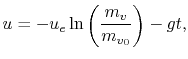where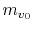is the initial mass of the rocket. We can also write this result as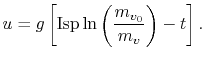We can view this equation as being similar to the Breguet Range Equation for aircraft. It presents the overall dependence of the principal performance parameter for a rocket (velocity,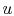), on the efficiency of the propulsion system (Isp), and the structural design (ratio of total mass to structural mass, since the initial mass is the fuel mass plus the structural mass and the final mass is only the structural mass).

Assuming the rate of fuel consumption is constant, the mass of the rocket varies over time as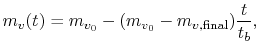whereis the time at which all of the propellant is used. This expression can be substituted into the equation for velocity and then integrated to find the height at the end of burnout: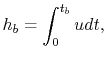which for a single stage sounding rocket with no drag and constant gravity yieldsThe final height of the rocket can then be determined by equating the kinetic energy of the vehicle at burnout with its change in potential energy between that point and the maximum height. This is left as an exercise for the reader.

To conclude this section, Figure 14.3 shows the most powerful rocket built, the NASA Saturn V rocket, which was used during the Apollo program to reach the Moon.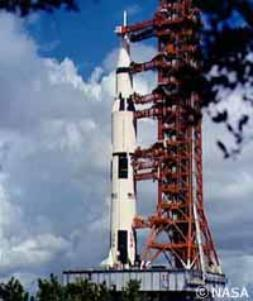UnifiedTP